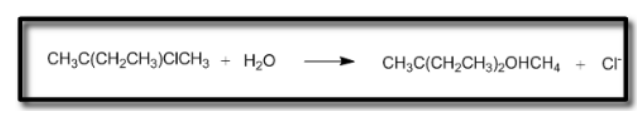# Problem: Consider the following reaction. Assuming no other changes, what would happen to the rate of the reaction if the concetration of the nucleophile was doubled and if the concentration of the alkyl halide was tripled? a. There would be no effect b. The rate would double c. The rate would triple d. The rate would increase 6-fold e. The rate would increase 9-fold

###### FREE Expert Solution
83% (329 ratings)
###### Problem Details

Consider the following reaction. Assuming no other changes, what would happen to the rate of the reaction if the concetration of the nucleophile was doubled and if the concentration of the alkyl halide was tripled?

a. There would be no effect

b. The rate would double

c. The rate would triple

d. The rate would increase 6-fold

e. The rate would increase 9-foldFrequently Asked Questions

What scientific concept do you need to know in order to solve this problem?

Our tutors have indicated that to solve this problem you will need to apply the Substitution Comparison concept. You can view video lessons to learn Substitution Comparison. Or if you need more Substitution Comparison practice, you can also practice Substitution Comparison practice problems.

What is the difficulty of this problem?

Our tutors rated the difficulty ofConsider the following reaction. Assuming no other changes, ...as high difficulty.

How long does this problem take to solve?

Our expert Organic tutor, Johnny took 5 minutes and 44 seconds to solve this problem. You can follow their steps in the video explanation above.

What professor is this problem relevant for?

Based on our data, we think this problem is relevant for Professor Ndonye's class at MONTGOMERY COLLEGE.